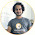## Wednesday, May 28, 2014

### Check if a number is Armstron or not.

Note- A number is armstrong if the sum of the cubes of digit of number is equal to the number.
ex- 407 = 4*4*4 + 0*0*0 + 7*7*7

import java.util.Scanner;

public class ArmstrongNum{
public static void main(String[] args){
Scanner in = new Scanner(System.in);
System.out.println("Enter a number which you want to check whether that is armstrong or not: ");
int n = in.nextInt();
int a = n, r=0, s=0;

while(a!=0){
r = a%10;
a = a/10;
s = s + r*r*r;
}
if(s==n){
System.out.println("Number "+n+" is an armstrong number.");
}else{
System.out.println("Number "+n+" is not an armstrong number.");
}
}
}

output

Enter a number which you want to check whether that is armstrong or not:
407
Number 407 is an armstrong number.

1.1.